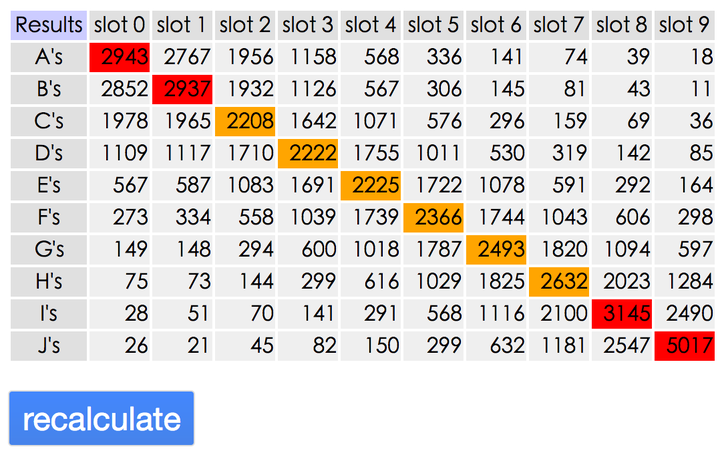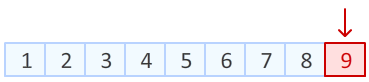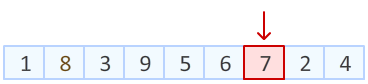# JavaScript数组乱序

1) 首先，毫无疑问： @顾轶灵 轶灵大佬给出的 Fisher–Yates shuffle 洗牌算法 是最完美乱序的算法/方法之一了，正解无疑。

2) 同时，很多答案提到了：

``[12,4,16,3].sort(function() {    return .5 - Math.random();});``

3) 也有答案提到了优秀的 lodash 库 `_.shuffle` 方法。这也是正解，事实上翻开 lodash 源码相关部分，这个方法正是采用了 Fisher–Yates shuffle 洗牌算法。感兴趣的同学可以进行参阅。

## 1) 为什么借助 sort 方法不是真正意义上的完全乱序？​

``var letters = ['A','B','C','D','E','F','G','H','I','J'];``

letters 这样一个数组使用 array.sort 方法进行了 10000 次乱序处理，并把乱序的每一次结果可视化输出。每个元素（ABCD...）出现的位置次数进行记录:v8 在处理 sort 方法时，使用了插入排序和快排两种方案。当目标数组长度小于10时，使用插入排序；反之，使用快排。

## 2) Fisher–Yates shuffle 洗牌算法是什么，为什么满足需求？​

Step1： 第一步需要做的就是，从数组末尾开始，选取最后一个元素。Step2：上一步中，我们已经把数组末尾元素进行随机置换。

Step3： 理解了前两部，接下来就是依次进行，如此简单。``Array.prototype.shuffle = function() {    var array = this;    var m = array.length,        t, i;    while (m) {        i = Math.floor(Math.random() * m--);        t = array[m];        array[m] = array[i];        array[i] = t;    }    return array;}``

note

``const random = Math.random;const shuffle = arr => arr.map(item => {value: item, key: random()}).sort((a, b) => b.key - a.key).map(item => item.value)``